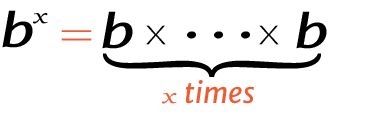# 4 raise to the power of 5

Place the base and exponent in the power calculator to get the right answer for value of 5^4, 54 (5 power 4), or 4 raised to the power of 5.

## How to calculate 5 raise to the power of 4?

This is how you can solve (5) 4 manually.

Solution:

Step 1: Simplify the data.

Base
ExponentStep 2: Multiply the 5 to itself 4 times.

Using this exponents calculator could be much easier to figure out an exponential expression.